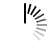# C Programming Examples

## C Program for Extended Euclidean algorithms

```#include <stdio.h>
int EuclideanExtended(int a, int b, int* x, int* y) {
if (a == 0) {
*x = 0;
*y = 1;
return b;
}
int xtemp, ytemp; // To store results of recursive call
int res = EuclideanExtended(b % a, a, &xtemp, &ytemp);
*x = ytemp - (b / a) * xtemp;
*y = xtemp;
return res;
}
int main() {
int x, y;
int a = 60, b = 25;
int res = EuclideanExtended(a, b, &x, &y);
printf("gcd(%d, %d) = %d", a, b, res);
}```

## C Program for Difference between sums of odd and even digits

```#include<stdio.h>
long isDiffZero(int n) {
if(n % 11 == 0){
return 1;
}
else{
return 0;
}
}
main() {
int n;
printf("Enter a number: ");
scanf("%d", &n);
if(isDiffZero(n)){
printf("Difference is zero");
}
else{
printf("Difference is non zero");
}
}```

## C Program for cube sum of first n natural numbers

```#include<stdio.h>
long cube_sum_n_natural(int n) {
long sum = 0;
int i;
for (i = 1; i <= n; i++) {
sum += i * i * i; //cube i and add it with sum
}
return sum;
}
main() {
int n;
printf("Enter value of n: ");
scanf("%d", &n);
printf("Result is: %ld", cube_sum_n_natural(n));
}```

## C Function Specifier Ex

```#include<stdio.h>
_Noreturn int myAdd(int a, int b){
return a + b;
}
main() {
int x = 10, y = 20;
printf("The value is: %d\n", myAdd(x, y));
}```

## C function specifier

```#include<stdio.h>
int myAdd(int a, int b){
return a + b;
}
main() {
int x = 10, y = 20;
printf("The value is: %d\n", myAdd(x, y));
}```

## C Generic keyword Ex

```#include<stdio.h>
#define INCREMENT(X) _Generic( (X), char: X+10, int: X+1, float: X+2.5, default: 0)
main() {
int x = 5; float y = 2.56; char z = 'A';
printf("Integer Increment: %d\n", INCREMENT(x));
printf("Float Increment: %f\n", INCREMENT(y));
printf("Character Increment: %c\n", INCREMENT(z));
}```

## C Generic keyword

```#include<stdio.h>
#define INCREMENT(X) ++X
main() {
int x = 5; float y = 2.56; char z = 'A';
printf("Integer Increment: %d\n", INCREMENT(x));
printf("Float Increment: %f\n", INCREMENT(y));
printf("Character Increment: %c\n", INCREMENT(z));
}```

## C Program for Egg Dropping Puzzle - DP-11

```#include<stdio.h>
#define MAX_VAL 9999
int max(int a, int b) {
return (a > b)? a: b;
}
int eggTrialCount(int eggs, int floors){ //minimum trials for worst case
int minTrial[eggs+1][floors+1]; //to store minimum trials for i-th egg and jth floor
int res, i, j, k;
for (i = 1; i <= eggs; i++){ //one trial to check from first floor, and no trial for 0th floor
minTrial[i] = 1;
minTrial[i] = 0;
}
for (j = 1; j <= floors; j++) //when egg is 1, we need 1 trials for each floor
minTrial[j] = j;
for (i = 2; i <= eggs; i++){ //for 2 or more than 2 eggs
for (j = 2; j <= floors; j++){ //for second or more than second floor
minTrial[i][j] = MAX_VAL;
for (k = 1; k <= j; k++){
res = 1 + max(minTrial[i-1][k-1], minTrial[i][j-k]);
if (res < minTrial[i][j])
minTrial[i][j] = res;
}
}
}
return minTrial[eggs][floors]; //number of trials for asked egg and floor
}
int main (){
int egg, maxFloor;
printf("Enter number of eggs: ");
scanf("%d", &egg);
printf("Enter max Floor: ");
scanf("%d", &maxFloor);
printf("Minimum number of trials: %d", eggTrialCount(egg, maxFloor));
}```

## C/C++ Function Call Puzzle

```#include<stdio.h>
void myFunction() {
printf("Function called\n");
}
int main() {
myFunction();
myFunction(2);
}```

## C Programming Language Puzzle

```#include<stdio.h>
#define MERGE(x, y) y##x
main(){
printf("%d", MERGE(48, 96));
}```

AdvertisementsWe use cookies to provide and improve our services. By using our site, you consent to our Cookies Policy.